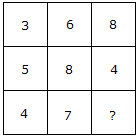# Verbal Reasoning - Character Puzzles - Discussion

### Discussion :: Character Puzzles - Character Puzzles 1 (Q.No.49)

49.

Which one will replace the question mark ?[A]. 6 [B]. 7 [C]. 8 [D]. 9

Explanation:

(5 + 3)/2 = 4

and (6 + 8)/2 = 7

Therefore (8 + 4)/2 = 6.

 Vicky said: (Jan 7, 2011) An another solution for this problem is.. 3+6+8=17 5+8+4=17 and therefore 4+7+6=17.

 Indhu said: (Aug 25, 2020) 3+6+8 = 17. 5+8+4 = 17. So, We will take 4+7+x = 17. x = 17-11. Which is equal to 6. Therefore it is 6.